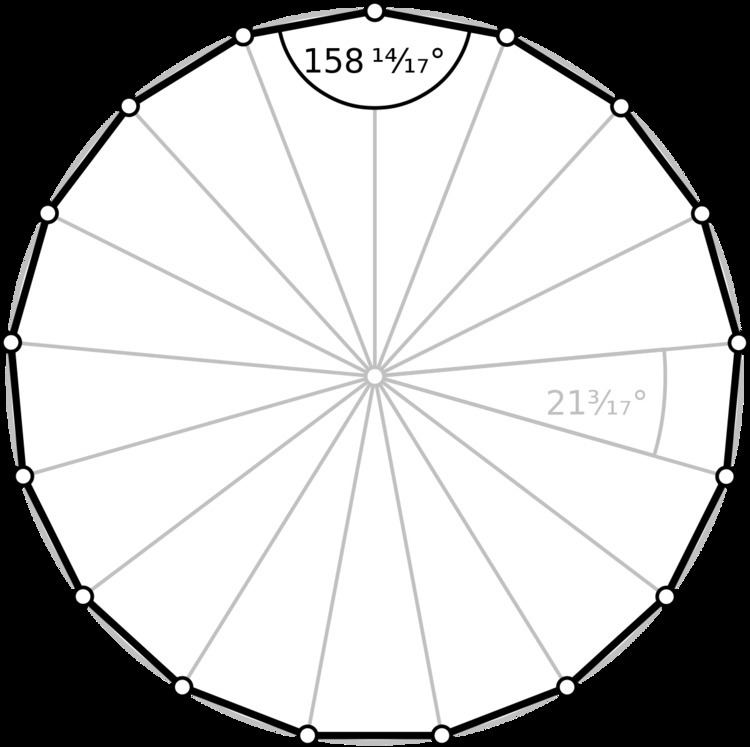# Heptadecagon

Updated on
 Type  Regular polygonSchläfli symbol  {17}Dual polygon  Self Edges and vertices  17Internal angle (degrees)  ≈158.82°Symmetry group  Dihedral (D17), order 2×17

In geometry, a heptadecagon or 17-gon is a seventeen-sided polygon.

## Regular heptadecagon

A regular heptadecagon is represented by the Schläfli symbol {17}.

## Construction

As 17 is a Fermat prime, the regular heptadecagon is a constructible polygon (that is, one that can be constructed using a compass and unmarked straightedge): this was shown by Carl Friedrich Gauss in 1796 at the age of 19. This proof represented the first progress in regular polygon construction in over 2000 years. Gauss's proof relies firstly on the fact that constructibility is equivalent to expressibility of the trigonometric functions of the common angle in terms of arithmetic operations and square root extractions, and secondly on his proof that this can be done if the odd prime factors of n are distinct Fermat primes, which are of the form F n = 2 2 n + 1 . Constructing a regular heptadecagon thus involves finding the cosine of 2 π / 17 in terms of square roots, which involves an equation of degree 17—a Fermat prime. Gauss' book Disquisitiones Arithmeticae gives this as (in modern notation):

16 cos 2 π 17 = 1 + 17 + 34 2 17 + 2 17 + 3 17 34 2 17 2 34 + 2 17 .

Constructions for the regular triangle, pentagon, pentadecagon, and polygons with 2h times as many sides had been given by Euclid, but constructions based on the Fermat primes other than 3 and 5 were unknown to the ancients. (The only known Fermat primes are Fn for n = 0, 1, 2, 3, 4. They are 3, 5, 17, 257, and 65537.)

The explicit construction of a heptadecagon was given by Herbert William Richmond in 1893. The following method of construction uses Carlyle circles, as shown below. Based on the construction of the regular 17-gon, one can readily construct n-gons with n being the product of 17 with 3 or 5 (or both) and any power of 2: a regular 51-gon, 85-gon or 255-gon and any regular n-gon with 2h times as many sides.

Another construction of the regular heptadecagon using straightedge and compass is the following:

The following simple design comes from Herbert William Richmond from the year 1893:

• The point N3 is very close to the center point of Thales' theorem over AF.
• Another more recent construction is given by Callagy.

## Symmetry

The regular heptadecagon has Dih17 symmetry, order 34. Since 17 is a prime number there is one subgroup with dihedral symmetry: Dih1, and 2 cyclic group symmetries: Z17, and Z1.

These 4 symmetries can be seen in 4 distinct symmetries on the heptadecagon. John Conway labels these by a letter and group order. Full symmetry of the regular form is r34 and no symmetry is labeled a1. The dihedral symmetries are divided depending on whether they pass through vertices (d for diagonal) or edges (p for perpendiculars), and i when reflection lines path through both edges and vertices. Cyclic symmetries in the middle column are labeled as g for their central gyration orders.

Each subgroup symmetry allows one or more degrees of freedom for irregular forms. Only the g17 subgroup has no degrees of freedom but can seen as directed edges.

## Related polygons

A heptadecagram is a 17-sided star polygon. There are seven regular forms given by Schläfli symbols: {17/2}, {17/3}, {17/4}, {17/5}, {17/6}, {17/7}, and {17/8}.

## Petrie polygons

The regular heptadecagon is the Petrie polygon for one higher-dimensional regular convex polytope, projected in a skew orthogonal projection:

## References

Similar Topics
Spendthrift (film)
Sandra Allen (artist)
Nguyễn Xuân Vinh
Topics